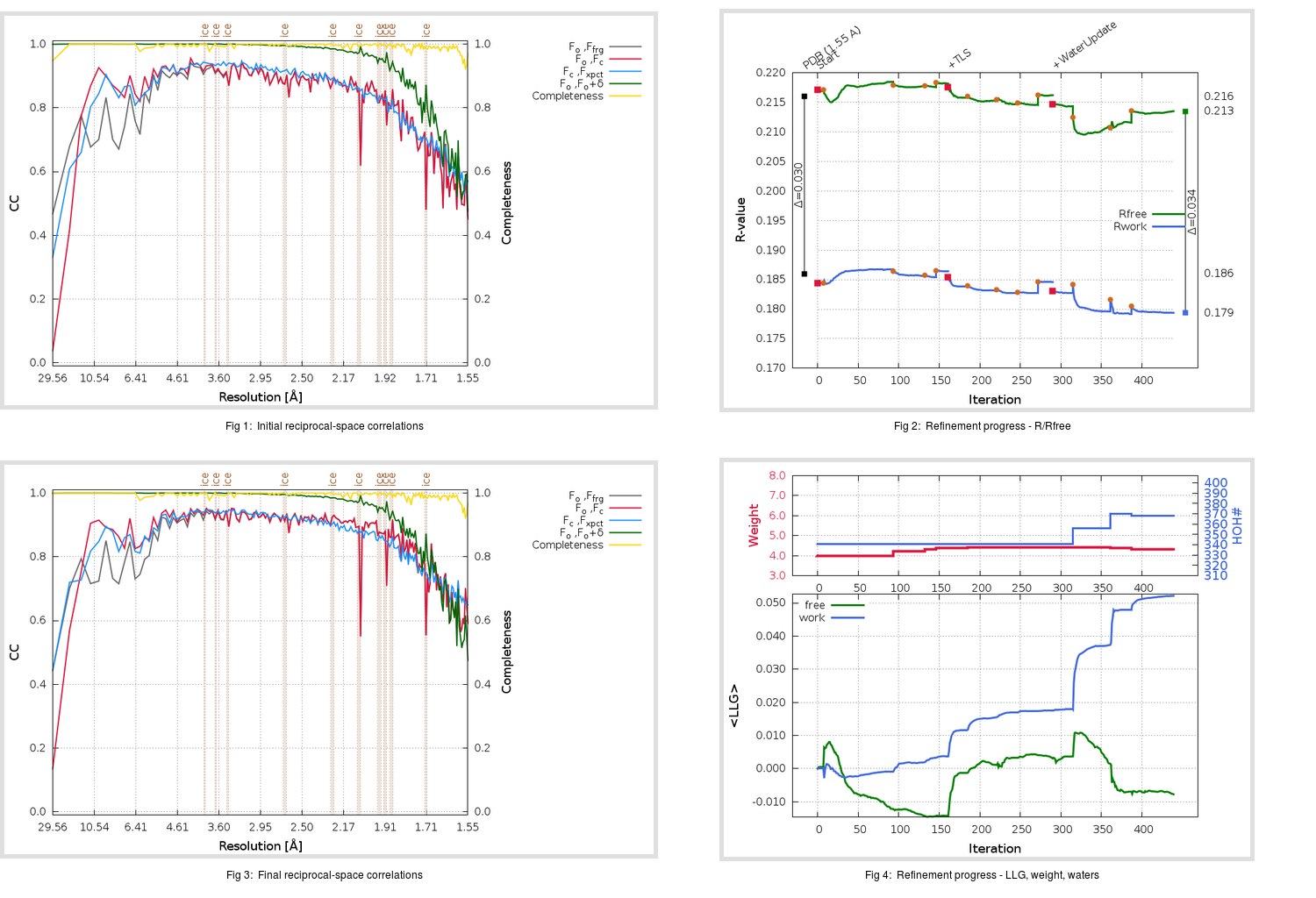Content:

## Deposited

` `
 Date deposited Date data collection Resolution R, Rfree 20200315 20200305 1.55 0.1840 0.2160

Molprobity (CCP4 7.0 version) summary:

```Ramachandran outliers =   0.33 %
favored =  98.01 %
Rotamer outliers      =   1.11 %
C-beta deviations     =     0
Clashscore            =   4.56
RMS(bonds)            =   0.0128
RMS(angles)           =   1.78
MolProbity score      =   1.27
Resolution            =   1.55
R-work                =   0.1840
R-free                =   0.2160
```

```Number of waters      =   341

<B> (all atoms) =   20.87 ( sd =    8.07 ) for       2786 non-hydrogen atoms
<B>   (protein) =   19.59 ( sd =    7.32 ) for       2416 non-hydrogen atoms
<B>     (water) =   29.93 ( sd =    8.67 ) for        341 non-hydrogen atoms
<B>    (others) =   26.96 ( sd =    8.78 ) for         29 non-hydrogen atoms

B min/max       (all non-hydrogen atoms) =    9.88 /  129.13
B min/max   (protein non-hydrogen atoms) =   10.31 /   57.79
B min/max     (water non-hydrogen atoms) =    9.88 /  129.13
B min/max     (other non-hydrogen atoms) =   19.56 /   35.35
```

## BUSTER (re-)refinement

` `

Molprobity (CCP4 7.0 version) summary:

```Ramachandran outliers =   0.33 %
favored =  98.68 %
Rotamer outliers      =   0.74 %
C-beta deviations     =     0
Clashscore            =   3.94
RMS(bonds)            =   0.0111
RMS(angles)           =   1.56
MolProbity score      =   1.18
Resolution            =   1.55
R-work                =   0.1794
R-free                =   0.2135
```

```Number of waters      =   368

<B> (all atoms) =   22.20 ( sd =    8.44 ) for       2813 non-hydrogen atoms
<B>   (protein) =   20.54 ( sd =    6.77 ) for       2416 non-hydrogen atoms
<B>     (water) =   32.93 ( sd =   10.64 ) for        368 non-hydrogen atoms
<B>    (others) =   27.22 ( sd =    9.56 ) for         29 non-hydrogen atoms

B min/max       (all non-hydrogen atoms) =    9.19 /   81.81
B min/max   (protein non-hydrogen atoms) =    9.19 /   59.24
B min/max     (water non-hydrogen atoms) =    9.57 /   81.81
B min/max     (other non-hydrogen atoms) =   16.52 /   33.59
```

Refinement progression:Results:

` `
 File Remark 5RFX_aB_refine.01_03_refine.pdb.gz exact refinement commands are in header 5RFX_aB_refine.01_03_refine.mtz.gz including original deposited data and several re-refinement map coefficients 5RFX_aB_refine.01_03_BUSTER_model.cif.gz including any non-standard compound restraints 5RFX_aB_refine.01_03_BUSTER_refln.cif.gz